# Math in Focus Grade 4 Chapter 6 Practice 6 Answer Key Renaming Whole Numbers when Adding and Subtracting Fractions

Practice the problems of Math in Focus Grade 4 Workbook Answer Key Chapter 6 Practice 6 Renaming Whole Numbers when Adding and Subtracting to score better marks in the exam.

## Math in Focus Grade 4 Chapter 6 Practice 6 Answer Key Renaming Whole Numbers when Adding and Subtracting Fractions

Fill in the missing numerators.

Example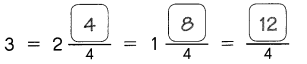Question 1.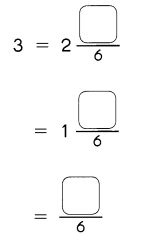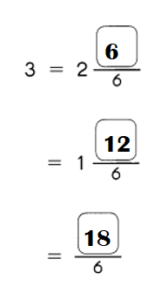Explanation:
3 = 2$$\frac{6}{6}$$ = 2 + 1.
= 1$$\frac{12}{6}$$ = 1 + (12)6 = 1 + 2.
= $$\frac{18}{6}$$

Question 2.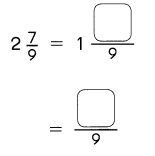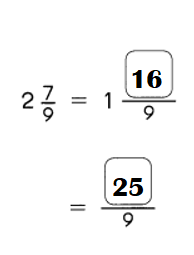Explanation:
2$$\frac{7}{9}$$ = 1$$\frac{16}{9}$$
= $$\frac{25}{9}$$

Question 3.
$$\frac{4}{9}$$ + $$\frac{2}{3}$$
$$\frac{4}{9}$$ + $$\frac{2}{3}$$ = 13 ÷ 9 or $$\frac{13}{9}$$

Explanation:
$$\frac{4}{9}$$ + $$\frac{2}{3}$$
= (4 + 6) ÷ 9
= 13 ÷ 9 or $$\frac{13}{9}$$

Question 4.
$$\frac{1}{6}$$ + $$\frac{11}{12}$$
$$\frac{1}{6}$$ + $$\frac{11}{12}$$ = 13 ÷ 12 or  $$\frac{11}{12}$$

Explanation:
$$\frac{1}{6}$$ + $$\frac{11}{12}$$
= (2 + 11) ÷ 12
= 13 ÷ 12 or  $$\frac{11}{12}$$

Question 5.
$$\frac{1}{4}$$ + $$\frac{3}{8}$$ + $$\frac{3}{4}$$
$$\frac{1}{4}$$ + $$\frac{3}{8}$$ + $$\frac{3}{4}$$ = 11 ÷ 8 or $$\frac{11}{8}$$

Explanation:
$$\frac{1}{4}$$ + $$\frac{3}{8}$$ + $$\frac{3}{4}$$
= (2 + 3) ÷ 8 + $$\frac{3}{4}$$
= $$\frac{5}{8}$$ + $$\frac{3}{4}$$
= [5 + 6] ÷ 8
= 11 ÷ 8 or $$\frac{11}{8}$$

Question 6.
$$\frac{4}{5}$$ + $$\frac{7}{10}$$ + $$\frac{9}{10}$$
$$\frac{4}{5}$$ + $$\frac{7}{10}$$ + $$\frac{9}{10}$$ = 12 ÷ 5 or $$\frac{12}{5}$$

Explanation:
$$\frac{4}{5}$$ + $$\frac{7}{10}$$ + $$\frac{9}{10}$$
= (8 + 7) ÷ 10 + $$\frac{9}{10}$$
= $$\frac{15}{10}$$ + $$\frac{9}{10}$$
= (15 + 9) ÷ 10
= $$\frac{24}{10}$$
= 12 ÷ 5 or $$\frac{12}{5}$$

Subtract. Express each answer as a mixed number in simplest form.

Example
2 – $$\frac{1}{3}$$
Method 1
2 – $$\frac{1}{3}$$ = $$\frac{2}{1}$$ – $$\frac{1}{3}$$
= $$\frac{6}{3}$$ – $$\frac{1}{3}$$
= $$\frac{5}{3}$$ = 1$$\frac{2}{3}$$

Metgod 2
2 – $$\frac{1}{3}$$ = 1$$\frac{3}{3}$$ – $$\frac{1}{3}$$
= 1$$\frac{2}{3}$$

Question 7.
3 – $$\frac{5}{6}$$ – $$\frac{1}{3}$$
3 – $$\frac{5}{6}$$ – $$\frac{1}{3}$$ = $$\frac{5}{2}$$

Explanation:
3 – $$\frac{5}{6}$$ – $$\frac{1}{3}$$
= 3 – [(5 – 2) ÷ 6]
= 3 – $$\frac{3}{6}$$
= (18 – 3) ÷ 6
= $$\frac{15}{6}$$
= $$\frac{5}{2}$$

Question 8.
2 – $$\frac{1}{4}$$ – $$\frac{1}{4}$$
2 – $$\frac{1}{4}$$ – $$\frac{1}{4}$$ = 2.

Explanation:
2 – $$\frac{1}{4}$$ – $$\frac{1}{4}$$
= 2 – (0)
= 2.

Question 9.
2 – $$\frac{2}{7}$$ – $$\frac{3}{14}$$
2 – $$\frac{2}{7}$$ – $$\frac{3}{14}$$ = $$\frac{27}{14}$$

Explanation:
2 – $$\frac{2}{7}$$ – $$\frac{3}{14}$$
= 2 – [(4 – 3) ÷ 14]
= 2 – $$\frac{1}{14}$$
= (28 – 1) ÷ 14
= $$\frac{27}{14}$$

Question 10.
3 – $$\frac{7}{10}$$ – $$\frac{3}{4}$$
3 – $$\frac{7}{10}$$ – $$\frac{3}{4}$$ = $$\frac{61}{20}$$
3 – $$\frac{7}{10}$$ – $$\frac{3}{4}$$
= 3 + $$\frac{1}{20}$$
= $$\frac{61}{20}$$